##Conic passing through four points and tangent to a line

Desargues involution theorem can be used to find the conic passing through four points and tangent to a given line. Assuming the four points {A,B,C,D} in general position, and the line (e) not passing through the four points or the points of intersection of opposite sides {E,F,G}, Desargues theorem asserts that the two intersection points of each conic-member of the conic bundle through {A,B,C,D} with (e) are in involution. The involution (f) depending only on the four points and the line.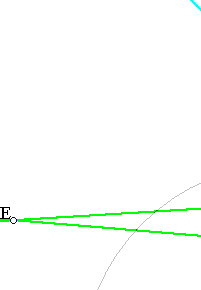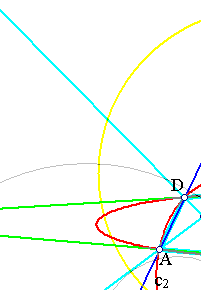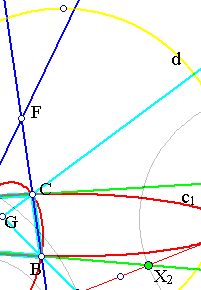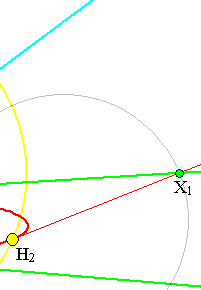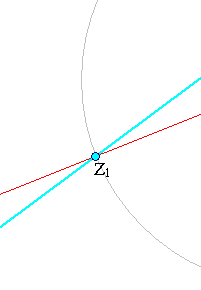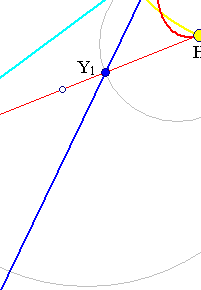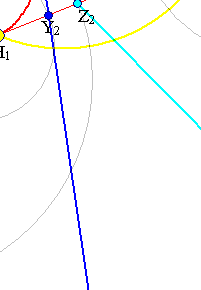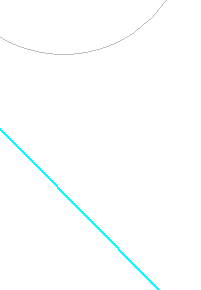The conics we are looking for pass through the fixed points of the involution f, hence there are either two or none solutions to the above problem. In the case f has two fixed points, they can be determined by drawing a circle d orthogonal to two circles with diameters defined by two pairs of conjugate points, such as (X1,X2), (Y1,Y2). The intersection points (if any) of (d) with line (e) are the fixed points of the involution f. This is explained in InvolutionBasic2.html .

CrossRatio0.html
DesarguesInvolution.html
FourPtsAndTangent2.html
HomographicRelation.html
HomographicRelationExample.html
Involution.html
InvolutionBasic.html
InvolutionBasic2.html

### References

Doerrie, Heinrich 100 Great Problems of Elementary Mathematics New York, Dover, 1965, p. 269.